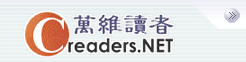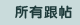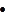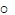设万维读者为首页广告服务技术服务联系我们关于万维简体繁体手机版
 首页 新闻 论坛 博客 视频 分类广告 购物版主：
 五 味 斋 茗香茶语 天下论坛 竞技沙龙 彩虹之约 摄友部落 诗词歌赋 七荤八素 高山流水 海 二 代 教育学术 笑林之声 健康生活 史地人物 军事天地 跨国婚姻 恋恋风尘 灵机一动 股市财经 加国移民 流行前线 新 大 陆 影视娱乐 焦点房谈 我爱我车 美国移民
 万维读者网 > 灵机一动 > 帖子
 讨论趣味数学279 送交者: zhf 2020年03月20日17:41:50 于 [灵机一动] 发送悄悄话 AB是圆O的切线，切点是A。D是圆O内的一点，DB与圆O相交于C。已知BC=DC=3，OD=2，AB=6，求圆O的半径。【图】设圆的半径为r。过D点做AB的垂线交于E点。令AE=x。过C点做AO的垂线交于F点。DE=sqrt(6^2-(6-x)^2)= sqrt(12x-x^2)     (1)AO=DE+sqrt(2^2-x^2)sqrt(12x-x^2) + sqrt(2^2-x^2) =r      (2)考虑三角形OCF，由勾股定理得((6+x)/2)^2+ (sqrt(12x-x^2)/2+sqrt(2^2-x^2))^2=r   (3)(2),(3)联立，经过悠长的推导得到58x^2-156x+81=0    (4)解(4)得到x=0.7，代入(2)得r=4.686。
 0%(0)
 0%(0)用圆的切割线定理做比较容易 - zhf 03/21/20 (5)高，实在是高。　　/无内容 - 零加一中 03/22/20 (0)利用圆的切割线定理比较容易做。r=√22　　/无内容 - gugeren 03/20/20 (1)实用资讯
 一周点击热帖 更多>>
 一周回复热帖
 历史上的今天：回复热帖
 2019: 趣味的数学 - 31 2019: 趣味的数学 - 30 2015: 范儿722——育儿（管不管）：恒心与自律